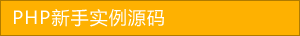﻿ PHP 与 Go 的语法区别 - php高级应用 - PHP粉丝网

# PHP 与 Go 的语法区别

##### 发布:smiling　来源: PHP粉丝网 　添加日期：2022-06-17 10:01:28　浏览: 评论：0

Go 是由 Google 设计的一门静态类型的编译型语言。它有点类似于 C，但是它包含了更多的优点，比如垃圾回收、内存安全、结构类型和并发性。它的并发机制使多核和网络机器能够发挥最大的作用。这是 GoLang 的最佳卖点之一。此外，Go 速度快，表现力强，干净且高效。这也是 Go 如此吸引开发者学习的原因。

PHP 是一种动态类型语言，它使新手更容易编写代码。现在的问题是，PHP 开发人员能否从动态类型语言切换到像 Go 这样的静态类型语言？为了找到答案，让我们对比一下 Go 和 PHP 之间的语法差异。

Go 同时支持有符号和无符号整数，而 PHP 只支持有符号整数。

Go 与 PHP 相比没有对象。但是，Go 有一个类似于 object 的 struct 类型。

PHP 数据类型:

boolean

string

integer // Signed integer, PHP does not support unsigned integers.

float (also known as "floats", "doubles", or "real numbers")

array

object

null

resource

Go 数据类型:

string

bool

int  int8  int16  int32  int64 // Signed integer

uint uint8 uint16 uint32 uint64 uintptr // Unsigned integers

byte // alias for uint8

rune // alias for int32

float32 float64

complex64 complex128

array

slices

map

struct

Go 使用 var 声明全局变量和函数变量。但是，它也支持带有初始化程序的简写语法，但只能在函数内部使用。另一方面，PHP 仅支持带有初始化程序的变量声明。

1. // 变量声明
2.
3. // Go               // PHP
4.
5. var i int           \$i = 0      // integer
6.
7. var f float64       \$f = 0.0    // float
8.
9. var b bool          \$b = false  // boolean
10.
11. var s string        \$s = ""     // string
12.
13. var a string     \$a = []     // array
14.
15. // 简短的变量声明
16.
17. // Go                      // PHP
18.
19. i := 0                     \$i = 0      // integer
20.
21. f := 0.0                   \$f = 0.0    // float
22.
23. b := false                 \$b = false  // boolean
24.
25. s := ""                    \$s = ""     // string
26.
27. a := string{"hello"}    \$a = []     // array

1. // Go
2.
3. i := 42             // Signed integer
4.
5. f := float64(i)     // Float
6.
7. u := uint(f)        // Unsigned integer
8.
9. // PHP
10.
11. \$i = 1;
12.
13. \$f = (float) \$i;    // 1.0
14.
15. \$b = (bool) \$f      // true
16.
17. \$s = (string) \$b    // "1"

1. // Go
2.
3. var a string
4.
5. a = "Hello"
6.
7. a = "World"
8.
9. // OR
10.
11. a := string{"hello""world"
12.
13. // PHP
14.
15. \$a = [
16.
17.     "hello"
18.
19.     "world"
20.
21. ];
22.
23. Maps
24.
25. // Go
26.
27. m := map[string]string{
28.
29.     "first_name""Foo"
30.
31.     "last_name""Bar"
32.
33.
34. // PHP
35.
36. \$m = [
37.
38.     "first_name" => "Foo"
39.
40.     "last_name" => "Bar"
41.
42. ];

Go 不支持对象。但是，您可以使用 structs 实现 object 之类的语法。

1. // Go
2.
3. package main
4.
5. import "fmt"
6.
7. type Person struct {
8.
9.     Name string
10.
12.
13.
14. func main() {
15.
16.     person := Person{"Foo bar""Sydney, Australia"
17.
18.     fmt.Println(person.Name)
19.
20.
21. // PHP
22.
23. \$person = new stdClass;
24.
25. \$person->Name = "Foo bar"
26.
28.
29. echo \$person->Name;
30.
31. // 或使用类型转换
32.
33. \$person = (object) [
34.
35.     'Name' => "Foo bar"
36.
38.
39. ];
40.
41. echo \$person->Name;

Go 和 PHP 函数之间的主要区别是； Go 函数可以返回任意数量的结果，而 PHP 函数只能返回一个结果。但是，PHP 可以通过返回数组来模拟相同的功能。

1. // Go
2.
3. package main
4.
5. import "fmt"
6.
7. func fullname(firstName string, lastName string) (string) {
8.
9.     return firstName + " " + lastName
10.
11.
12. func main() {
13.
14.     name := fullname("Foo""Bar"
15.
16.     fmt.Println(name)
17.
18.
19. // PHP
20.
21. function fullname(string \$firstName, string \$lastName) : string {
22.
23.     return \$firstName . " " . \$lastName
24.
25.
26. \$name = fullname("Foo""Bar");
27.
28. echo \$name
29.
30. // 返回多个结果
31.
32. // Go
33.
34. package main
35.
36. import "fmt"
37.
38. func swap(x, y string) (string, string) {
39.
40.     return y, x
41.
42.
43. func main() {
44.
45.     a, b := swap("hello""world"
46.
47.     fmt.Println(a, b)
48.
49.
50. // PHP
51.
52. // 返回一个数组以获得多个结果
53.
54. function swap(string \$x, string \$y): array {
55.
56.     return [\$y\$x];
57.
58.
59. [\$a\$b] = swap('hello''world');
60.
61. echo \$a\$b

If-Else

1. // Go
2.
3. package main
4.
5. import (
6.
7.     "fmt"
8.
9.
10. func compare(a int, b int) {
11.
12.     if a > b {
13.
14.         fmt.Println("a is bigger than b"
15.
16.     } else {
17.
18.         fmt.Println("a is NOT greater than b"
19.
20.     }
21.
22.
23. func main() {
24.
25.     compare(12, 10);
26.
27.
28. // PHP
29.
30. function compare(int \$a, int \$b) {
31.
32.     if (\$a > \$b) {
33.
34.         echo "a is bigger than b"
35.
36.     } else {
37.
38.         echo "a is NOT greater than b"
39.
40.     }
41.
42.
43. compare(12, 10);
44.
45. Switch

Go 的 switch 与 C，C+，Java，JavaScript 和 PHP 中的类似，除了 Go 只运行选中的 case，而不是随后的所有 case。 实际上， break 语句在这些语言中的每个 case 后都是必需的，而在 Go 中则是自动补充的。另一个重要的区别是 Go 的 switch cases 不需要是常量，并且涉及的值也不必是整数。

1. // Go
2.
3. package main
4.
5. import (
6.
7.     "fmt"
8.
9.     "runtime"
10.
11.
12. func main() {
13.
14.     fmt.Print("Go runs on "
15.
16.     os := runtime.GOOS;
17.
18.     switch os {
19.
20.     case "darwin"
21.
22.         fmt.Println("OS X."
23.
24.     case "linux"
25.
26.         fmt.Println("Linux."
27.
28.     default
29.
30.         fmt.Printf("%s.\n", os)
31.
32.     }
33.
34.
35. // PHP
36.
37. echo "PHP runs on "
38.
39. switch (PHP_OS) {
40.
41.     case "darwin"
42.
43.         echo "OS X."
44.
45.         break
46.
47.     case "linux"
48.
49.         echo "Linux."
50.
51.         break
52.
53.     default
54.
55.         echo PHP_OS;
56.
57.
58. For 循环
59.
60. // Go
61.
62. package main
63.
64. import "fmt"
65.
66. func main() {
67.
68.     sum := 0
69.
70.     for i := 0; i < 10; i++ {
71.
72.         sum += i
73.
74.     }
75.
76.     fmt.Println(sum)
77.
78.
79. // PHP
80.
81. \$sum = 0;
82.
83. for (\$i = 0; \$i < 10; \$i++) {
84.
85.     \$sum += \$i
86.
87.
88. echo \$sum

While 循环

Go 自身没有 while 循环的语法。相应的，Go 使用 for 循环代替实现 while 循环.

1. // Go
2.
3. package main
4.
5. import "fmt"
6.
7. func main() {
8.
9.     sum := 1
10.
11.     for sum < 100 {
12.
13.         sum += sum
14.
15.     }
16.
17.     fmt.Println(sum)
18.
19.
20. // PHP
21.
22. \$sum = 1;
23.
24. while (\$sum < 100) {
25.
26.     \$sum += \$sum
27.
28.
29. echo \$sum
30.
31. Foreach/Range

PHP 使用 foreach 迭代数组和对象。与之对应，Go 使用 range 迭代 slice 或 map。

1. // Go
2.
3. package main
4.
5. import "fmt"
6.
7. func main() {
8.
9.     colours := []string{"Maroon""Red""Green""Blue"
10.
11.     for index, colour := range colours {
12.
13.         fmt.Printf("index: %d, colour: %s\n", index, colour)
14.
15.     }
16.
17.
18. // PHP
19.
20. \$colours = ["Maroon""Red""Green""Blue"];
21.
22. foreach(\$colours as \$index => \$colour) {
23.
24.     echo "index: {\$index}, colour: {\$colour}\n"
25.

## 相关文章